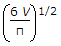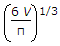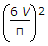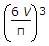# Online Mechanical Engineering Test - Machine Design Test 2Loading Test...

Instruction:

• This is a FREE online test. DO NOT pay money to anyone to attend this test.
• Total number of questions : 20.
• Time alloted : 30 minutes.
• Each question carry 1 mark, no negative marks.
• DO NOT refresh the page.
• All the best :-).

1.

A locking device extensively used in marine type connecting rod ends is a

A.
 jam nutB.
 castle nutC.
 sawn nutD.
 ring nut2.

The V-belts are particularly suitable for __________ drives.

A.
 shortB.
 long3.

The ratio of driving tensions in V-belt drives is __________ flat belt drives.

A.
 equal toB.
 sin α times more thanC.
 sin α times less thanD.
 cosec α times more than4.

Which of the following has threads at both ends?

A.
 BoltB.
 Tap boltC.
 StudD.
 none of these5.

A screw is specified by its

A.
 major diameterB.
 minor diameterC.
 pitch diameterD.
 pitch6.

A thin spherical shell of internal diameter d is subjected to an internal pressure p. If V is the storage capacity of shell, then its diameter will be

A.B.C.D.7.

Two rigid plates are clamped by means of bolt and nut with an initial force F. After tightening, a separating force P (P < F) is applied to the lower plate, which in turn acts on the nut. The tension in the both after this is

A.
 F + PB.
 F - PC.
 PD.
 F8.

The shear stress at a point in a shaft subjected to a torque is

A.
 directly proportional to the polar moment of inertia and to the distance of the point from the axisB.
 directly proportional to the applied torque and inversely proportional to the polar moment of inertiaC.
 directly proportional to the applied torque and the polar moment of inertiaD.
 inversely proportional to the applied torque and the polar moment of inertia9.

A bolt is designed on the basis of __________ with a large factor of safety.

A.
 direct tensile stressB.
 direct compressive stressC.
 direct bending stressD.
 direct shear stress10.

When a closely coiled helical spring is subjected to a couple about its axis, the stress induced in the wire material of the spring is

A.
 bending stress onlyB.
 a combination of torsional shear stress and bendingC.
 direct shear stress onlyD.
 a combination of bending stress and direct shear stress11.

In radial bearings, the load acts __________ to the axis of rotation.

A.
 parallelB.
 perpendicular12.

Which of the following statement is correct ?

A.
 The cold rolled shafting is stronger than hot rolled shaftingB.
 The hot rolled shafting is stronger than cold rolled shaftingC.
 The cold rolled and hot rolled shafting are equally strongD.
 The shafts are not made by rolling process13.

In a turnbuckle, if one of the rods has left hand threads, then the other rod will have

A.
 right hand threadsB.
 left hand threadsC.
 multiple threadsD.
 pointed threads14.

A hollow shaft will transmit a __________ torque than a solid shaft of the same mass and same material.

A.
 smallerB.
 greater15.

When a cylinder is subjected to a very high internal fluid pressure, the thin cylinder should be used.

A.
 CorrectB.
 Incorrect16.

The pitch diameter is the __________ diameter of an external or internal screw thread.

A.
 effectiveB.
 smallestC.
 largest17.

The diameter of knuckle pin in a knuckle joint is usually taken as (where d = Diameter of the rod)

A.
 0.5 dB.
 0.8 dC.
 dD.
 1.2 d18.

When carbon in the cast iron is in the form of cementite, the cast iron will be of white colour.

A.
 CorrectB.
 Incorrect19.

The strength of a riveted joint is equal to

A.
 tearing strength of plate (Pt)B.
 shearing strength of rivet (Ps)C.
 crushing strength of rivet (Pc)D.
 least value of Pt Ps and Pc20.

The V-belt is used to transmit great amount of power and when the two pulleys are very near to each other.

A.
 CorrectB.
 Incorrect